Open in App
Not now

# Rock, Paper, Scissor game – Python Project

• Difficulty Level : Easy
• Last Updated : 29 Nov, 2022

In this article, we will see how we can create a rock paper and scissor game using Tkinter. Rock paper scissor is a hand game usually played between two people, in which each player simultaneously forms one of the three shapes with an outstretched hand. These shapes are “rock”, “paper”, and “scissors”.

## Game Winner Conditions

Let there be a Player who is playing with a computer as an opponent.  Now,

• If the player selects Paper and Computer Selects Scissor – Computer wins
• If the player selects Rock and Computer Selects Scissor – Player 1 wins
• If the player selects Paper and Computer Selects Rock – Player 1 wins
• And If the player selects Paper and Computer Selects Paper – Draw
• If the player selects Rock and Computer Selects Rock – Draw
• If the player selects Scissor and Computer Selects Scissor – Draw

## Tools and Technologies Used

• Tkinter: It is a standard Python interface to the Tk GUI toolkit shipped with Python. Python with tkinter is the fastest and easiest way to create GUI applications.
• Random: Python defines a set of functions that are used to generate or manipulate random numbers through the random module.
• PIL: Python Imaging Library (expansion of PIL) is the de facto image processing package for Python language. It incorporates lightweight image processing tools that aids in editing, creating and saving images.

## GUI Implementation

### Part 1:Image processing and handling

1) Import Python Module Tkinter to execute GUI applications.

2) From PIL Import ImageTk, Image for image processing.

3) Import random which will help the computer to select options rock, paper, or scissor randomly.

4) Now an object is created root which is the main window object.

• Title of this window -> Rock Paper Scissor
• Dimensions of this window -> “800×680”

5) Create canvas of width=800, height=680

6) Now we Create labels on GUI window

• l1 =Player-> font=’Algerian’, size=25
• l2 =Computer-> font=’Algerian’, size=25
• l3 =Vs font=’Algerian’, size=40

7) Now labels are placed on window

• l1 at x=80, y=20
• l2 at x=560, y=20
• l3 at x=370, y=230

For Default Image:

1) An variable named img_p is used to open the default hand image and resize it to (300,300). This will be shown at default condition in the game at the place of the player.

2) An variable named img_c is used to store flipped default image from left to right using transpose function and it is saved in the variable. This will be shown at default condition in the game at the place of the computer’s side.

3) Images img_p and img_c are loaded on the canvas now using Tk.PhotoImage

For Rock Image:

1) An variable named rock_p is used to open the rock hand image and resize it to (300,300). This will be shown at the player’s side when the player selects rock in the game.

2) An variable named rock_c is used to store flipped rock hand image from left to right using transpose function and will be shown at computer’s side when the computer randomly selects rock in the game.

3) Images rock_p and  rock_c are loaded on the canvas now using Tk.PhotoImage.

For Paper Image

1) An variable named paper_p is used to open the paper hand image and resize it to (300,300). This will be shown at the player’s side when the player selects paper in the game.

2) An variable named paper_c is used to store flipped paper hand images from left to right using the transpose function and will be shown at the computer’s side when the computer randomly selects paper in the game.

3) Images paper_p and  paper_c is loaded on the canvas now using Tk.PhotoImage.

For Scissors Image

1) An variable named scissor_p is used to open the scissor hand image and resize it to (300,300). This will be shown at player’s side when player selects scissor in the game.

2) An variable named scissor_c is used to store flipped scissor hand image from left to right using transpose function and will be shown at computer’s side when computer randomly selects scissor in the game.

3) Images scissor_p and  scissor_c are loaded on the canvas now using Tk.PhotoImage.

For Selection Image:

1) An variable named img_s is used to open the selection of hand images i.e. Combined image of Rock, Paper & Scissor, and resize it to (300,130).

2) Image img_s is loaded on the canvas now using Tk.PhotoImage.

### Part 2: Game Implementation

1) A game function is defined in which we have a list named select having values 1, 2, 3 representing rock, paper, and scissors respectively.

2) Here random.choice randomly selects options 1-3 for computer

3) Set image for Player on canvas

• If Player selects 1 (Rock)  Show rock image on canvas using create_image.
• If Player selects 2 (Paper) Show paper image on canvas using create_image.
• If Player selects 3 (Scissor) Show scissor image on canvas using create_image.

4) Set image for Computer on canvas

• If Computer selects 1 (Rock)  Show rock image on canvas using create_image.
• If Computer selects 2 (Paper) Show paper image on canvas using create_image.
• If Computer selects 3 (Scissor) Show scissor image on canvas using create_image.

5) Obtaining the result

• If Player chooses Rock and computer chooses Rock OR If the player chooses Paper and the computer chooses Paper OR If the player chooses Scissor and the computer chooses Scissor. Result Shown-> Draw
• If the Player chooses Rock and computer choose Scissor OR If the player chooses Paper and computer choose Rock OR If the player chooses Scissor and computer choose Paper.  Result Shown -> Player won
• Else Result Shown-> Computer won

Note: This result is shown on canvas in form of text having dimensions (390,600), font=’Algerian’, tag=’result’.

6) Buttons

• Clear Button -> Deletes the present result and switches the figures at both sides to its default conditions.
• Rock  Button -> Selects choice 1 in function game and Shows an Image of Hand showing Rock On Player side.
• Paper Button -> Selects choice 2 in function game and Shows Image of Hand showing Paper On Player side
• Scissor Button -> Selects choice 3 in function game and Shows an Image of Hand showing the Scissor On the Player side.

Images Used: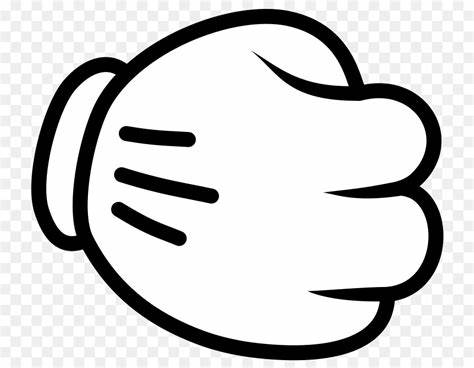default.jpg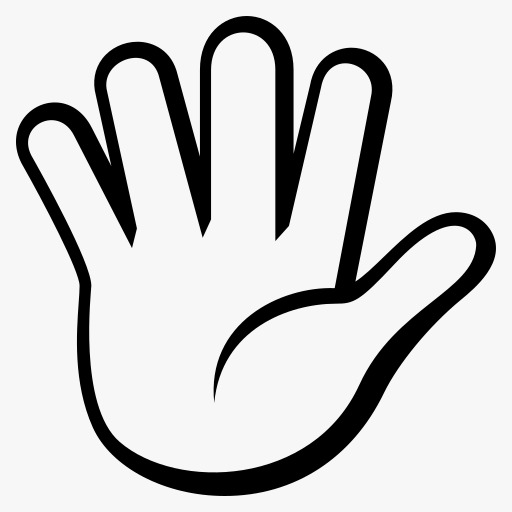paper.jpg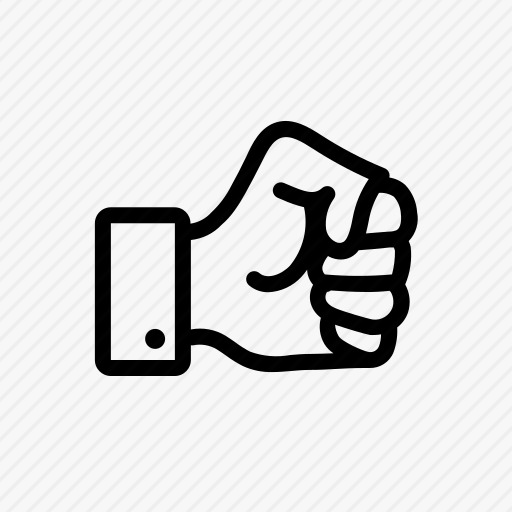rock.jpg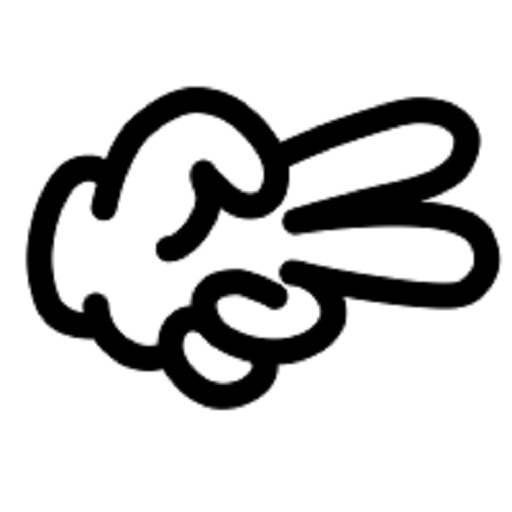scissor.jpg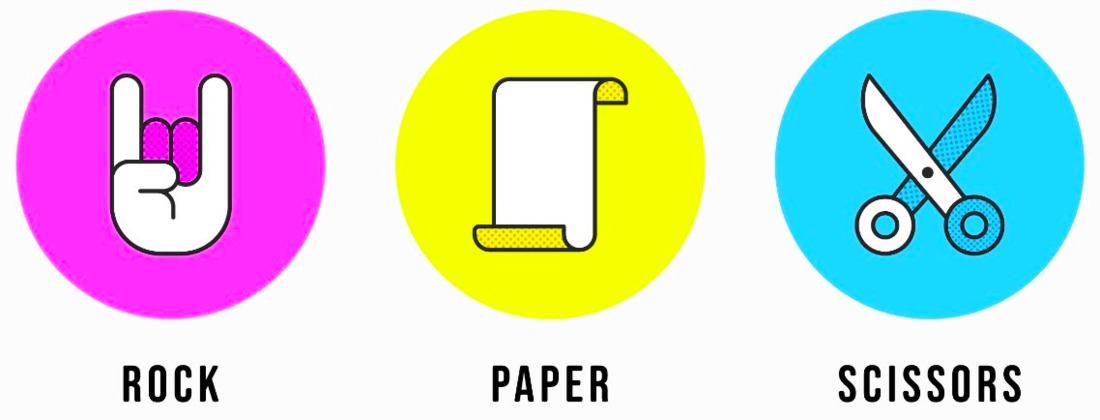Selection.jpg

main.py

## Python3

 `from` `tkinter ``import` `*` `from` `PIL ``import` `ImageTk, Image` `import` `random`   `# main window object` `root ``=` `Tk()`   `# Title of GUI window` `root.title(``'Rock Paper Scissor'``)`   `# Size of window` `root.geometry(``'800x680'``)`   `# Creating canvas` `canvas ``=` `Canvas(root, width``=``800``, height``=``680``)` `canvas.grid(row``=``0``, column``=``0``)`   `# Creating labels on GUI window` `l1 ``=` `Label(root, text``=``'Player'``, font``=``(``'Algerian'``, ``25``))` `l2 ``=` `Label(root, text``=``'Computer'``, font``=``(``'Algerian'``, ``25``))` `l3 ``=` `Label(root, text``=``'Vs'``, font``=``(``'Algerian'``, ``40``))`   `# Placing all the labels on window` `l1.place(x``=``80``, y``=``20``)` `l2.place(x``=``560``, y``=``20``)` `l3.place(x``=``370``, y``=``230``)`   `# Default image` `img_p ``=` `Image.``open``(``"default.jpeg"``)` `img_p ``=` `img_p.resize((``300``, ``300``))`   `# Flipping image from left to right` `img_c ``=` `img_p.transpose(Image.FLIP_LEFT_RIGHT)`   `# Loading images to put on canvas` `img_p ``=` `ImageTk.PhotoImage(img_p)` `img_c ``=` `ImageTk.PhotoImage(img_c)`   `# Rock image` `rock_p ``=` `Image.``open``(``'rock.jpeg'``)` `rock_p ``=` `rock_p.resize((``300``, ``300``))`   `# Flipping image from left to right` `rock_c ``=` `rock_p.transpose(Image.FLIP_LEFT_RIGHT)`   `# Loading images to put on canvas` `rock_p ``=` `ImageTk.PhotoImage(rock_p)` `rock_c ``=` `ImageTk.PhotoImage(rock_c)`   `# Paper image` `paper_p ``=` `Image.``open``(``'paper.jpeg'``)` `paper_p ``=` `paper_p.resize((``300``, ``300``))`   `# Flipping image from left to right` `paper_c ``=` `paper_p.transpose(Image.FLIP_LEFT_RIGHT)`   `# Loading images to put on canvas` `paper_p ``=` `ImageTk.PhotoImage(paper_p)` `paper_c ``=` `ImageTk.PhotoImage(paper_c)`   `# Scissor image` `scissor_p ``=` `Image.``open``(``'scissor.jpeg'``)` `scissor_p ``=` `scissor_p.resize((``300``, ``300``))`   `# Flipping image from left to right` `scissor_c ``=` `scissor_p.transpose(Image.FLIP_LEFT_RIGHT)`   `# Loading images to put on canvas` `scissor_p ``=` `ImageTk.PhotoImage(scissor_p)` `scissor_c ``=` `ImageTk.PhotoImage(scissor_c)`   `# Selection image` `img_s ``=` `Image.``open``(``"Selection.jpeg"``)` `img_s ``=` `img_s.resize((``300``, ``130``))` `img_s ``=` `ImageTk.PhotoImage(img_s)`   `# Putting image on canvas on specific coordinates` `canvas.create_image(``0``, ``100``, anchor``=``NW, image``=``img_p)` `canvas.create_image(``500``, ``100``, anchor``=``NW, image``=``img_c)` `canvas.create_image(``0``, ``400``, anchor``=``NW, image``=``img_s)` `canvas.create_image(``500``, ``400``, anchor``=``NW, image``=``img_s)`     `# game function` `def` `game(player):` `    ``select ``=` `[``1``, ``2``, ``3``]` `    `  `    ``# Randomly selects option for computer` `    ``computer ``=` `random.choice(select)`   `    ``# Setting image for player on canvas` `    ``if` `player ``=``=` `1``:` `      `  `        ``# Puts rock image on canvas` `        ``canvas.create_image(``0``, ``100``, anchor``=``NW, image``=``rock_p)` `    ``elif` `player ``=``=` `2``:` `        `  `        ``# Puts paper image on canvas` `        ``canvas.create_image(``0``, ``100``, anchor``=``NW, image``=``paper_p)` `    ``else``:` `        `  `        ``# Puts scissor image on canvas` `        ``canvas.create_image(``0``, ``100``, anchor``=``NW, image``=``scissor_p)`   `    ``# Setting image for computer on canvas` `    ``if` `computer ``=``=` `1``:` `        `  `        ``# Puts rock image on canvas` `        ``canvas.create_image(``500``, ``100``, anchor``=``NW, image``=``rock_c)` `    ``elif` `computer ``=``=` `2``:` `        `  `        ``# Puts paper image on canvas` `        ``canvas.create_image(``500``, ``100``, anchor``=``NW, image``=``paper_c)` `    ``else``:` `        `  `        ``# Puts scissor image on canvas` `        ``canvas.create_image(``500``, ``100``, anchor``=``NW, image``=``scissor_c)`   `    ``# Obtaining result by comparison` `    ``if` `player ``=``=` `computer:  ``# Case of DRAW` `        ``res ``=` `'Draw'` `        `  `    ``# Case of player's win` `    ``elif` `(player ``=``=` `1` `and` `computer ``=``=` `3``) ``or` `(player ``=``=` `2` `and` `computer ``=``=` `1``) ``or` `    ``(player ``=``=` `3` `and` `computer ``=``=` `2``):` `        ``res ``=` `'You won'` `    `  `    ``# Case of computer's win` `    ``else``:` `        ``res ``=` `'Computer won'`   `    ``# Putting result on canvas` `    ``canvas.create_text(``390``, ``600``, text``=``'Result:- '` `+` `res,` `                       ``fill``=``"black"``, font``=``(``'Algerian'``, ``25``), tag``=``'result'``)`     `# Function for clear button` `def` `clear():` `  `  `    ``# Removes result from canvas` `    ``canvas.delete(``'result'``)`   `    ``# Puts default image on canvas` `    ``canvas.create_image(``0``, ``100``, anchor``=``NW, image``=``img_p)` `    ``canvas.create_image(``500``, ``100``, anchor``=``NW, image``=``img_c)`     `# Button for selecting rock` `rock_b ``=` `Button(root, text``=``'Rock'``, command``=``lambda``: game(``1``))` `rock_b.place(x``=``35``, y``=``487``)`   `# Button for selecting paper` `paper_b ``=` `Button(root, text``=``'Paper'``, command``=``lambda``: game(``2``))` `paper_b.place(x``=``128``, y``=``487``)`   `# Button for selecting scissor` `scissor_b ``=` `Button(root, text``=``'Scissor'``, command``=``lambda``: game(``3``))` `scissor_b.place(x``=``220``, y``=``487``)`   `# Button for clear` `clear_b ``=` `Button(root, text``=``'CLEAR'``, font``=``(``'Times'``, ``10``, ``'bold'``),` `                 ``width``=``10``, command``=``clear).place(x``=``370``, y``=``28``)`   `root.mainloop()`

Output

Code Explanation:

1. The code starts by creating a window object called root.
2. The window has a title, which is “Rock Paper Scissor”.
3. The size of the window is set to 800×680 pixels.
4. Next, a canvas object is created.
5. The canvas has the same width and height as the window.
6. The grid command is used to create a grid on the canvas.
7. This will help us keep our drawings organized and easy to read.
8. The labels are then created using the text command.
9. Two labels are created – one for rock and one for paper.
10. These labels will be used in our game later on.
11. Finally, images are loaded into the canvas using the ImageTk library.
12. A random image is loaded for each player in our game (rock, paper, scissors).
13. The code creates a window and sets its title.
14. The window’s size is then set to 800×680 pixels.
15. Next, a canvas object is created and its grid attribute is set to row=0, column=0.
16. Finally, two labels are created and placed on the GUI window.
17. The code starts by creating three labels – Player, Computer, and Vs.
18. The first two labels are placed at the top-left corner of the window, while the third label is placed in the middle of the window.
19. Next, the code sets up some variables to store information about each label.
20. l1 and l2 are assigned a Label object with the name ‘Player’ and ‘Computer’, respectively.
21. l3 is assigned a Label object with the name ‘Vs’.
22. The next line creates an Image object named img_p and saves it to disk as a JPEG file.
23. The image size is set to 300×300 pixels.
24. Finally, img_p is initialized as an Image object.
25. The code creates three labels on the window, Player, Computer, and Vs.
26. The first label, l1, is placed at the top-left corner of the window and has a text of “Player” assigned to it.
27. The second label, l2, is placed in the middle of the window and has a text of “Computer” assigned to it.
28. The last label, l3, is placed at the bottom-right corner of the window and has a text of “Vs” assigned to it.
29. Next, the code opens an image named default.jpeg in Image object and sets its size to 300×300 pixels.
30. Finally, the code assigns this image as the default image for all three labels.
31. The code starts by loading the three images that will be used in the example.
32. The first image is a rock, the second is a paper, and the third is a selection image.
33. Next, the code flips each of these images so that they are displayed in reverse order.
34. This is done using the transpose() function.
35. The final step is to load each of these flipped images into an ImageTk PhotoImage object.
36. This allows us to display them onscreen together as one unit.
37. The code will first load the images “rock.jpeg”, “paper.jpeg”, and “scissor.jpeg” into the variables img_p, img_c, and scissor_p, respectively.
38. Next, the code will transpose each image’s left to right (LTR) direction so that they will be displayed on the canvas in their original orientation (RTL).
39. Finally, the code will create a new PhotoImage object for each image and assign it to the respective variables img_s and img_c.
40. The code first creates three images on the canvas.
41. The first image is at coordinates (0, 100), the second image is at coordinates (500, 100), and the third image is at coordinates (0, 400).
42. Next, the code defines a game function that will be used to control the player.
43. This game function takes one parameter – a player object – which represents a person in the game world.
44. The game function first checks to see if the player has moved within its bounds.
45. If not, it returns immediately.
46. Otherwise, it sets up two variables – x and y – to represent where on the screen the player currently is.
47. It then calls another function called draw() which will be used to draw all of the graphics in this game.
48. The draw() function first sets up an anchor point for each of its three images using create_anchor().
49. Next, it draws each of those images using create_image().
50. Finally, it assigns these values back to their respective variables so that they can be used by other parts of this program later on.
51. The code creates three images on the canvas, each at a specific coordinate.
52. The first image is at (0, 100), the second image is at (500, 100), and the third image is at (0, 400).
53. The game function will be called once for each of the three images.
54. In this function, we will ask the player to guess which of the three images they are looking at.
55. If they guess correctly, then the game is over and they win; if not, then we keep going.
56. The code starts by creating two variables, player and computer.
57. The player variable stores the value of 1, while the computer variable stores the value of 2.
58. Next, the code randomly selects one of the three options from the select list.
59. In this case, it randomly selects option 1.
60. The next line sets up an image for player on canvas using create_image().
61. The first argument is 0 (the x-coordinate), which specifies that the image should be placed at the bottom-left corner of the canvas.
62. The second argument is 100 (the y-coordinate), which specifies that the image should be centered in the canvas.
63. The third and fourth arguments are anchors (NW for north, NE for northeast, SW for southwest, and SE for southeast), which specify where on the canvas to place this image relative to other images on this canvas.
64. Finally, you provide a string representing your image filename.
65. Next, if player equals 1 (which means that we’re dealing with a rock object), then we use create_image() again but with a different set of arguments: anchor=WEST and image=rock_r.
66. This creates an image representing a rock object with its top facing westward.
67. If computer
68. The code will randomly select one of the three images (rock, paper, or scissor) to be displayed on the player’s canvas and also display the corresponding image for the computer.
69. The code begins by checking to see if the player is equal to or greater than the computer.
70. If so, then the code sets the result to “Draw”.
71. If not, then the code checks to see if the player is equal to or less than the computer.
72. If so, then the code sets the result to “You won”.
73. Finally, if neither of these conditions are met, then the code sets the result to “Computer won”.
74. The first condition checks whether player 1 is equal to 3 and computer 3.
75. The second condition checks whether player 2 is equal to 1 and computer 1.
76. The third condition checks whether player 3 is equal to 2 and computer 2.
77. If any of these comparisons are true, then that particular outcome will be set as the result.
78. The code compares the player’s number (1, 2, or 3) with the computer’s number (3, 2, or 1).
79. If the player equals the computer, then the result is set to “You won.”
80. If the player is different from the computer, then the code checks to see if the computer is winning.
81. If so, then the result is set to “Computer won.”
82. Otherwise, the code sets the result to “Draw.”
83. The code starts by creating a few variables.
84. The first is text, which will hold the message that is displayed on the screen.
85. Next, the code creates two buttons: rock_b and clear.
86. The rock_b button has a command attribute, which defines what action should be taken when it is clicked.
87. In this case, the command() function will be executed when the button is clicked.
88. This function takes one parameter—the number 1—and it will determine whether or not to play the game.
89. If you run this code now, you’ll see that clicking on the rock_b button results in a change in the message displayed onscreen (it now says “Result:- 0”).
90. The clear() function simply removes all of the content from the canvas object.
91. Finally, there are two images created using create_image().
92. The first image (img_p) will be placed at coordinates (0, 100), and its anchor point will be set to NW.
93. The second image (img_c) will be placed at coordinates (500, 100), and its anchor point will also be set to NW.
94. The code creates a button named rock_b on the root canvas.
95. The code defines the button as having the text Rock and a command that will run the game() function when clicked.
96. Next, the code creates two images on the canvas.
97. The first image is located at (0, 100) and has an anchor set to NW.
98. The second image is located at (500, 100) and also has an anchor set to NW.
99. Finally, the code defines a function named clear() that will remove all of the content from the canvas and replace it with default images.
100. The code in this example creates a three-button panel.
101. The first button is labeled “Paper,” and when it is clicked, the game() function is called.
102. This function takes two arguments: the number of turns that have passed since the last time the game was played, and the player’s current score.
103. The second button is labeled “Scissor.”
104. When it is clicked, the game() function is called again, but this time with an additional argument: the number of pieces left on the player’s side of the board.
105. If there are no more pieces left on that side of the board, then game over!
106. The third button is labeled “Clear.”
107. When it is clicked, all buttons and text in the panel are cleared (including any text that has been entered into those buttons by users).
108. The code creates a button named ‘paper_b’ and a button named ‘scissor_b’.
109. The first button will be activated when the player clicks on it, while the second button will be activated when the player presses down on it.
110. Finally, the last button is used to clear the screen.
111. When creating these buttons, you should keep in mind that they should be placed at different locations on the screen so that they are easy to access.
112. For example, paper_b should be placed at (x=128, y=487), while scissor_b should be placed at (x=220, y=487).

My Personal Notes arrow_drop_up
Related Articles